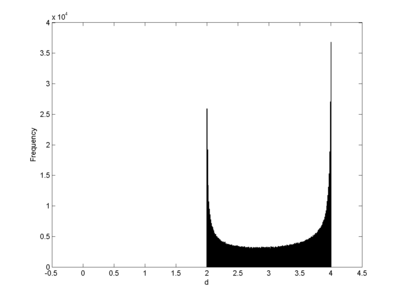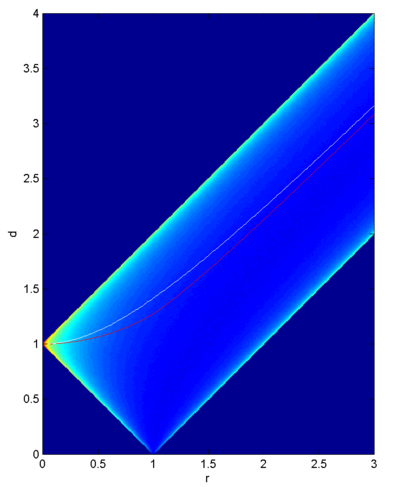## September 19, 2013

### Distance between planets

A question that came up recently: what is the average distance between two planets orbiting a star at radius R1 and R2 in circular orbits?

If we rescale things in units of R1, so planet 1 orbits at distance 1 and planet 2 at distance r=(R2/R1), the answer for the average is =(1/2pi) times the integral from 0 to 2 pi of sqrt((cos(x)-r)^2+sin(x)) dx... which unfortunately is only expressible in terms of the elliptic function of the second kind. Possible to calculate, but messy and hard to understand:
=(1/Pi) (Sqrt[(-1 + r)^2] EllipticE[-((4 r)/(-1 + r)^2)] + Sqrt[(1 + r)^2] EllipticE[(4 r)/(1 + r)^2])
Even implementing this calculation in software that has the function in the library needs some care, it is easy to slip up with some term or modulus somewhere.

However, a quick Monte Carlo simulation gives some nice results. First, the distribution has more probability mass close to the extremes than near the middle. Here is how it looks when r=3:Most of the time the planet is either on the far side or the close side of the sun by the reckoning of the other planet. So the average distance is somewhat misleading in the first place: the mode (the most probable point) is that it is on the other side, but the distribution is properly bimodal.

Plotting the distribution as a function of r gives the following nice picture:The colors denote probability density. For r=0 it is all located at distance 1. Then the possible range increases linearly, until r=1 and it briefly becomes possible for the planets to coincide (maybe not the most physical situation). As r becomes larger the distribution is all housed in the strip [r-1,r+1].

The red and white line indicate the median and mean, respectively. As we can see, they are not terribly representative of where the other planet actually is.

It turns out that the median curve can be approximated surprisingly well by 1+r^2/(1+r). The absolute error starts out as 0 for r=0, reaches a maximum of 0.1 for r=1 and then declines.

For large r the probability distribution becomes more symmetric converges to the arcsine distribution. The median and mean converge to r.

So a good guesstimate for the median distance is R1*(1+(R2/R1)^2/(1+(R2/R1))) = R1 + R2^2/(R1 + R2). It is not exact, but given that the actual distance is likely to be R1 away from it anyway, it is good enough for many purposes.

Posted by Anders3 at September 19, 2013 02:26 PM
Comments